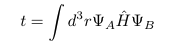# Outputting the hopping parameters (i.e. elements of the Hamiltonian)?

Hi,

is it possible to output the full Hamiltonian Matrix during a calculation? I ask because I want to get access to hopping parameters of my molecule and those are the elements of the Hamiltonian (see image).

Also, can I choose what orbitals to run a calculation with? For instance, can I choose to only run the s-orbitals available in my molecule for that?Can I ask what your background is in and what kinds of systems you’re interested in? It sounds like you’re interested in toy systems and are not a chemist by training.

I have a physics background but one of my projects deals with a peptide where I need to know the Hamiltonian of my system for a given set of orbitals. But before I get to that I want to know how to get the Hamiltonian for the simplest case. And the simplest case for me would be the Hubbard chain.

I don’t know what you mean by “know the Hamiltonian of my system for a given set of orbitals.” For any set of orthonormal orbitals, the molecular Hamiltonian is given as a linear combination of second quantized operators, with expansion coefficients given by one and two-electron integrals. Are you asking how you can get a program to compute the integrals for you? Are you trying to learn how to compute the integrals “from scratch”?

Psi is designed for molecular systems and does not support a Hubbard chain.

The “only s-orbital” approximation is not even qualitatively correct for most atoms. For hydrogen atoms, it’s qualitatively correct. Choose a STO-3G or STO-6G basis set, which is the bare minimum.

Forming the Hamiltonian matrix for a molecule is prohibitively expensive and is only ever done for pedagogical purposes. See here for an example.

The s orbital was referring to H2. What are the inputs for that case for instance?

This sounds like a question of basic Psi4 syntax. If you want to learn how to write an input file to compute the energy of H2 in the STO-3G basis set, please consult the many sample inputs we have available and read the manual.

If you still have a question after that, ask again.

I did the H2 example for the STO-3G basis. Reading through the manual I can see that what I want is probably given by the keyword HEFF_PRINT. But how do I set it to true? I did ‘set psimrcc HEFF_PRINT TRUE’ but that didn’t output me the Hamiltonian. Any ideas?

EDIT: I think I got it. Setting
Escf, scf_wfn = energy(‘SCF’, molecule=h2o, return_wfn=True)
scf_wfn.H().print_out()

I managed to print out the one-electron Hamiltonian.

`set psimrcc heff_print true` will only affect the PSIMRCC module, which I assure you is not what you want.

`scf_wfn.H()` is what quantum chemists call the core Hamiltonian. It is the matrix representation of the one-electron part of the Hamiltonian acting on the one-electron space. It does not represent the two-electron part of the Hamiltonian or wavefunctions of more than two electrons.

If you really want the Hamiltonian, see the link I included in my previous post.

And for the record, I still don’t know what you actually want. Do you want numerical values for matrix elements of the Hamiltonian between two-electron states of H2? Do you want formulas for those numerical values expressed in terms of integrals? Do you want formulas for the integrals?

I want the numerical values for matrix elements of the Hamiltonian between two-electron states.

Then yes, go look at the FCI pilot implementation I linked earlier.

You’ll need to change the molecule from H2O to H2, but that should be straightforward.